# Unit of Resistance

## Unit of Resistance:

Unit of Resistance – When a current flows in a material, the free electrons move through the material and collide with other atoms. These collisions cause the electrons to lose some of their energy. This loss of energy per unit charge is the drop in potential across the material. The amount of energy lost by the electrons is related to the physical property of the material. These collisions restrict the movement of electrons. The property of a material to restrict the flow of electrons is called resistance, denoted by R. The symbol for the resistor is shown in Fig. 1.3.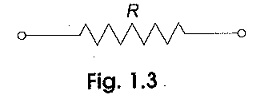The unit of resistance is ohm (Ω). Ohm is defined as the resistance offered by the material when a current of one ampere flows between two terminals with one volt applied across it.

According to Ohm’s law, the current is directly proportional to the voltage and inversely proportignal to the total resistance of the circuit, i.e.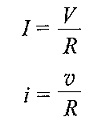We can write the above equation in terms of charge as follows.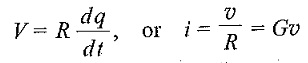where G is the conductance of a conductor. The units of resistance and conductance are ohm (Ω) and mho (∪) respectively.

When current flows through any resistive material, heat is generated by the collision of electrons with other atomic particles. The power absorbed by the resistor is converted to heat. The power absorbed by the resistor is given by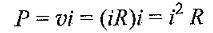where i is the current in the resistor in amps, and v is the voltage across the resistor in volts. Energy lost in a resistance in time t is given by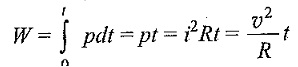where

v is the volts

R is in ohms

t is in seconds and

W is in joules

Updated: September 29, 2017 — 10:24 pm

`Please enable JavaScript in your browser to complete this form.Name *FirstLastEmail *Paragraph TextEmail`
` `